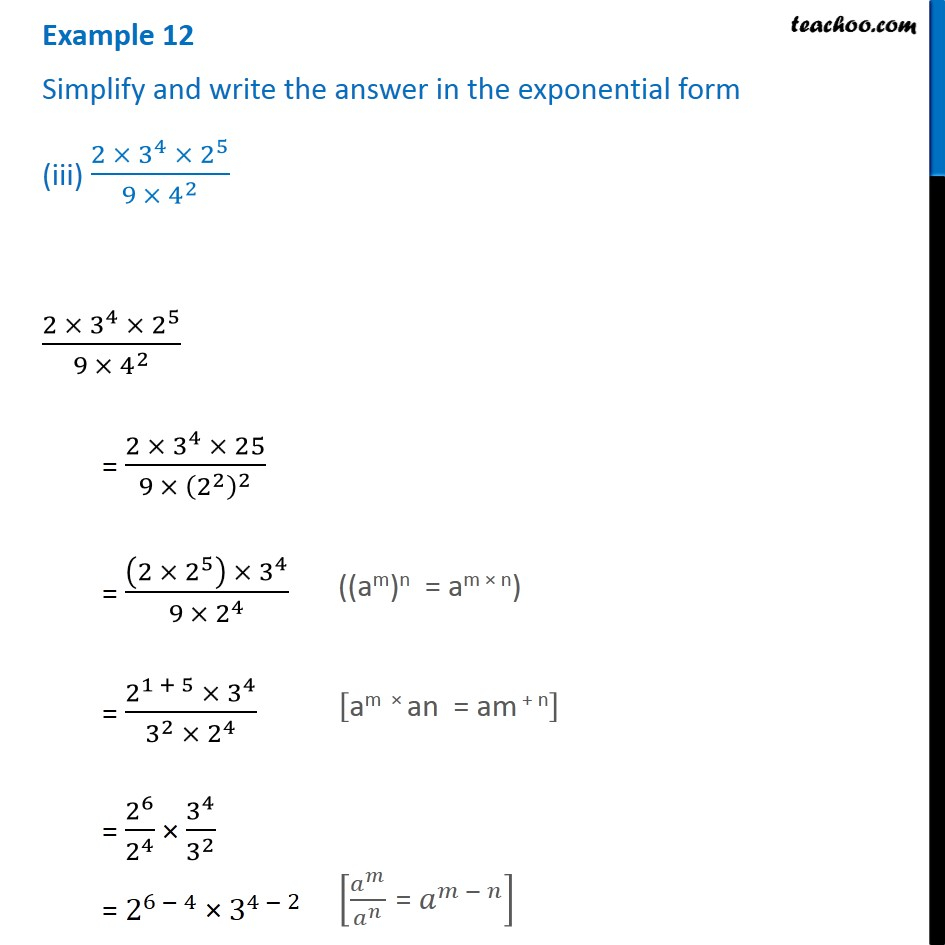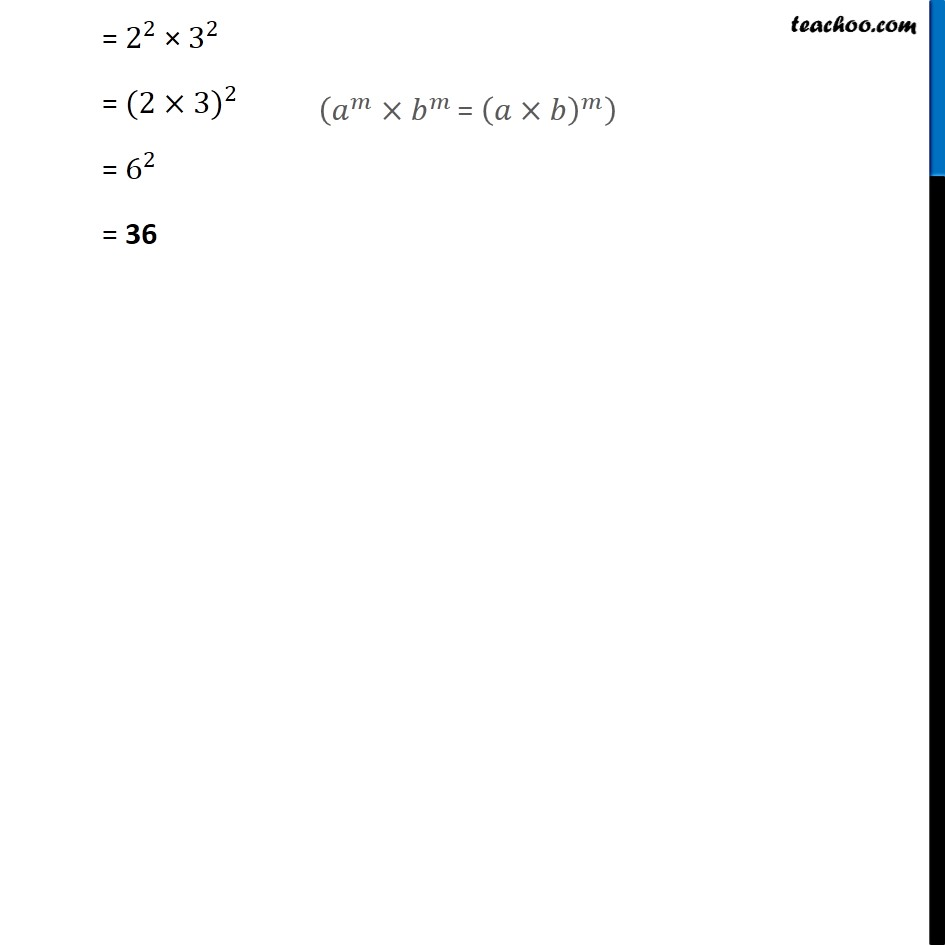1. Chapter 13 Class 7 Exponents and Powers
2. Serial order wise
3. Examples

Transcript

Example 12 Simplify and write the answer in the exponential form (iii) (2 × 3^4 × 2^5)/(9 ×〖 4〗^2 ) (2 × 3^4 × 2^5)/(9 × 4^2 ) = (2 × 3^4 × 25)/(9 ×〖 (2^2 )〗^2 ) = ((2 × 2^5 ) × 3^4)/(9 × 2^4 ) = (2^(1 + 5) × 3^4)/(3^2 × 2^4 ) = 2^6/2^4 × 3^4/3^2 = 2^(6 − 4) × 3^(4 − 2) ((am)n = am × n) ((am)n = am × n) [𝑎^𝑚/𝑎^𝑛 " = " 𝑎^(𝑚 − 𝑛) ] = 2^2 × 3^2 = 〖(2×3)〗^2 = 6^2 = 36 (𝑎^𝑚×𝑏^𝑚 " = " (𝑎×𝑏)^𝑚 )